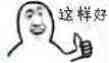# 博客

## Goodbye Dingyou题解

2018-02-18 18:10:43 By WuHongxun

### 新年的叶子

#### 算法三

$$\frac{\binom{k}{i}\times \binom{n-i-1}{n-k-1}\times \binom{n-k}{1}\times(k-i)!\times(n-k-1)!\times (i)!}{n!}\times (\sum_{j=i+1}^{n}\frac{m}{j})$$

#### 算法四

from WuHongxun### 算法四

#### 算法五

1. 当 $a_{i,j}+p_j-p_i\le -1$ 时，约束总能满足，不考虑该约束；
2. 当 $a_{i,j}+p_j-p_i\ge 1$ 时，约束不可能满足，该情况的概率直接返回 $0$；
3. 当 $a_{i,j}+p_j-p_i=0$ 时，约束等价于 $q_i\ge q_j$。

### 新年的代码

#### 算法一

$n \leq 10$？

#### 算法二dp就只需要$f_{i,j,k}$表示前$i$位处理完了，第$i$位先变成$j$让我们来继续操作后面的位，然后操作完后面的位之后我们把第$i$位从$S_i$变成了$k$，我们再回来用前面的位把它变成$T_i$的最小步数。

myy（matthew99）丢给了我一个他猜完结论后的这题代码，虽然我没看懂https://paste.ubuntu.com/p/6P7XZ6zT2W/

#### 算法三$f_{i,a,b}$表示dp完了前$i$个位置，在操作$i..n$位的过程中，需要第$i$个位置从$a$变成$b$的最小步数。### 新年的投票

#### 算法二## 评论

AntiLeaf

pw384

oscar

Thinkwice

AntiLeaf

jjikkollp

huangsi

lastans7
@WuHongxun 请问E题的算法四中，是任意一个k次多项式都可以对应一个解吗？
Enemy

suncongbo